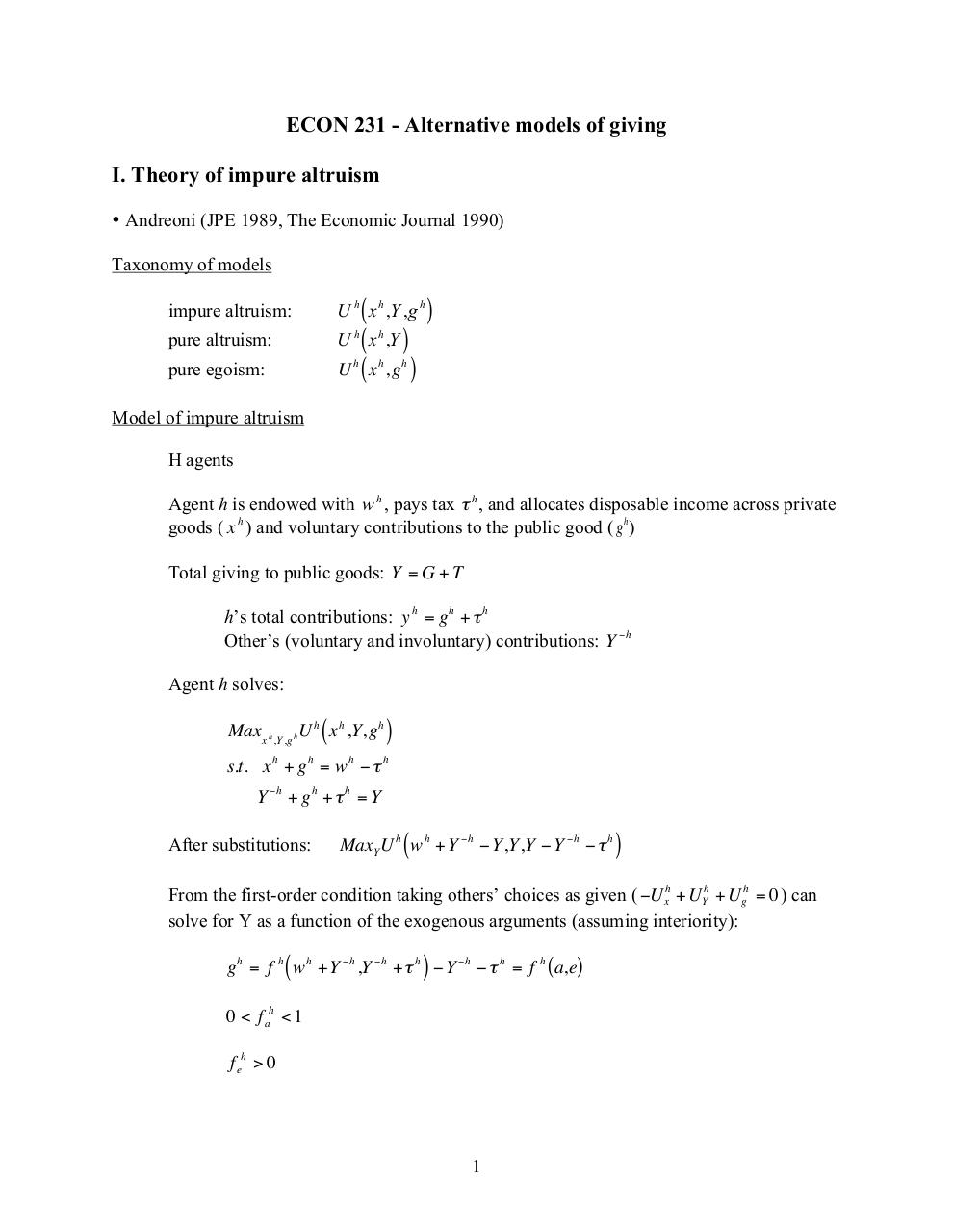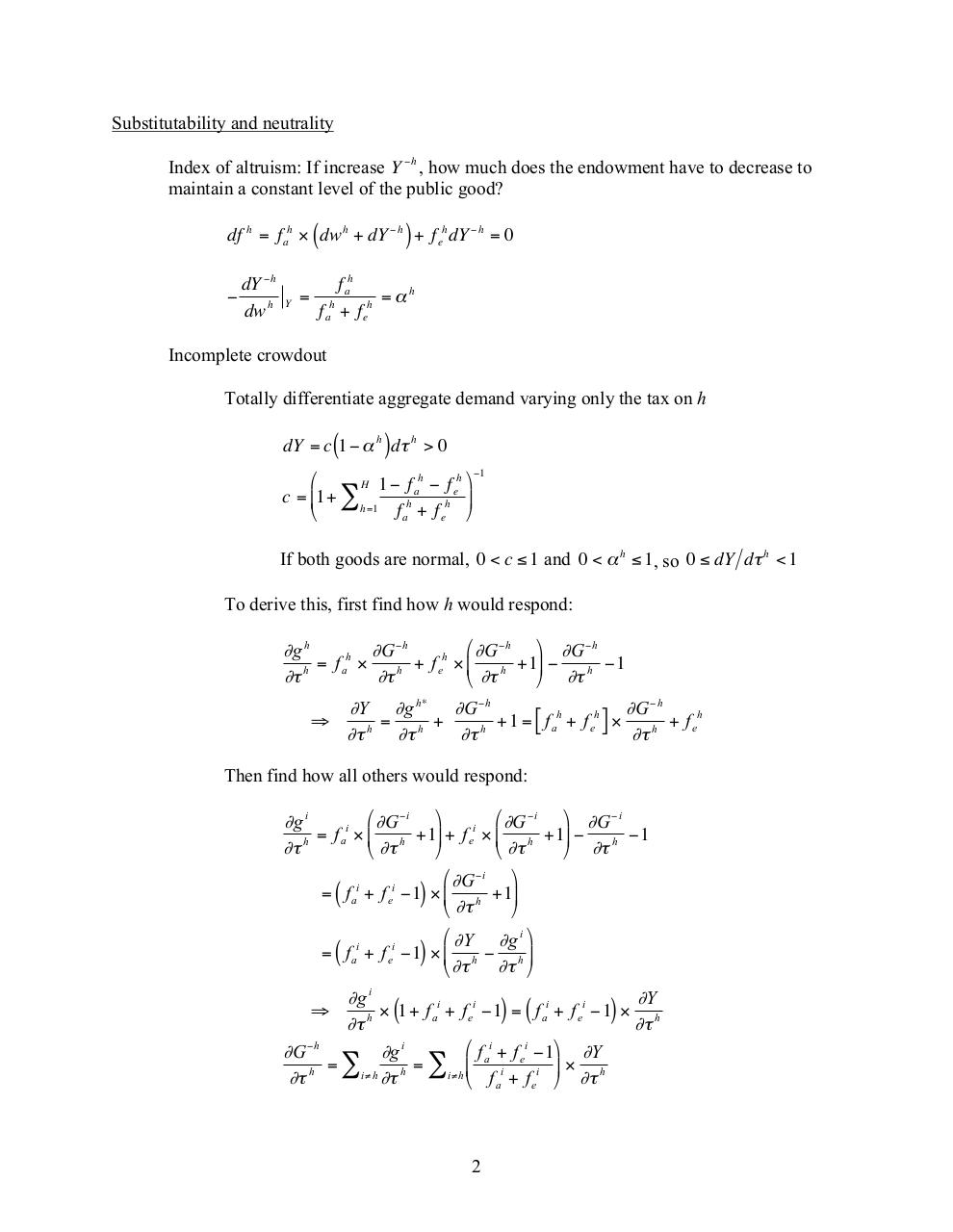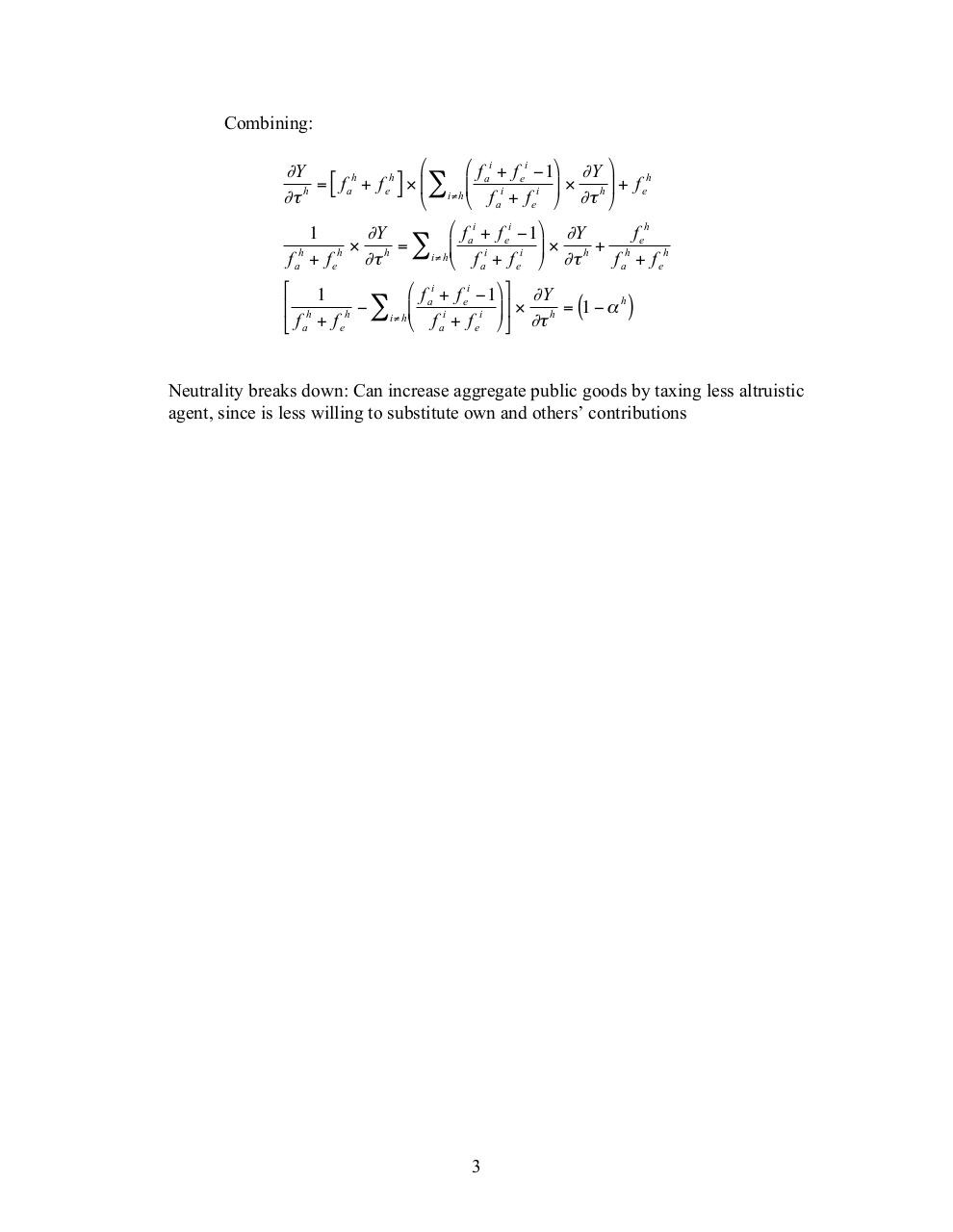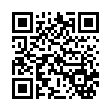# ec231 glow HO .pdf

### File information

Original filename: ec231_glow_HO.pdf
Title: ec231_glow_HO
Author: Julianne Cullen

This PDF 1.3 document has been generated by Microsoft Word / Mac OS X 10.6.8 Quartz PDFContext, and has been sent on pdf-archive.com on 18/02/2016 at 23:56, from IP address 128.54.x.x. The current document download page has been viewed 441 times.
File size: 459 KB (3 pages).
Privacy: public file

ec231_glow_HO.pdf (PDF, 459 KB)

### Document preview

ECON 231 - Alternative models of giving
I. Theory of impure altruism
• Andreoni (JPE 1989, The Economic Journal 1990)
Taxonomy of models
impure altruism:

U h ( x h ,Y ,g h )

pure altruism:

U h ( x h ,Y )
U h ( x h ,g h )

pure egoism:

Model of impure altruism

H agents

Agent h is endowed with w h , pays tax τ h , and allocates disposable income across private
goods ( x h ) and voluntary contributions to the public good ( g h )
Total giving to public
goods:€Y = G + T

h’s total contributions: y h = g h + τ h
Other’s (voluntary
and involuntary) contributions: Y −h

Agent h solves:

Max x h ,Y ,g h U h ( x h ,Y,g h )
s.t. x h + g h = w h − τ h
Y −h + g h + τ h = Y

After substitutions:

MaxY U h ( w h + Y −h − Y,Y,Y − Y −h − τ h )

From the first-order condition taking others’ choices as given ( −U xh + UYh + U gh = 0 ) can
solve for Y as
€ a function of the exogenous arguments (assuming interiority):
g h = f h ( w h + Y −h ,Y −h + τ h ) − Y −h − τ h = f h ( a,e)

0 &lt; f ah &lt; 1

f eh &gt; 0

1

Substitutability and neutrality
Index of altruism: If increase Y −h , how much does the endowment have to decrease to
maintain a constant level of the public good?
df h = f ah × (€dw h + dY −h ) + f eh dY −h = 0

dY −h
dw h

Y

=

f ah
= αh
f ah + f eh

Incomplete crowdout

Totally differentiate aggregate demand varying only the tax on h
dY = c (1 − α h ) dτ h &gt; 0
h
h ⎞ −1
⎛
H 1− f
a − fe
c = ⎜1+ ∑
⎟
h =1
f ah + f eh ⎠
⎝

If both goods are normal, 0 &lt; c ≤ 1 and 0 &lt; α h ≤ 1, so 0 ≤ dY dτ h &lt; 1

To derive this, first find how h would respond:

⎛ ∂G −h ⎞ ∂G −h €
∂g h
∂G −h
h
h
= fa ×
+ f e × ⎜ h +1⎟ −
h −1
∂τ h
∂τ h
⎝ ∂τ
⎠ ∂τ

∂Y ∂g h* ∂G −h
∂G −h
h
h
=
+
+1
=
f
+
f
×
[ a e ] ∂τ h + f eh
∂τ h ∂τ h
∂τ h

Then find how all others would respond:

⎛ ∂G −i ⎞
⎛ ∂G −i ⎞ ∂G −i
∂g i
i
i
=
f
×
+1
+
f
×
⎜ h
⎟ e ⎜ h +1⎟ −
a
h −1
∂τ h
⎝ ∂τ
⎠
⎝ ∂τ
⎠ ∂τ
⎛ ∂G −i ⎞
= ( f ai + f ei −1) × ⎜ h +1⎟
⎝ ∂τ
⎠
⎛ ∂Y ∂g i ⎞
i
i
= ( f a + f e −1) × ⎜ h − h ⎟
∂τ ⎠
⎝ ∂τ

∂g i
∂Y
i
i
i
i

h × (1+ f a + f e −1) = ( f a + f e −1) ×
∂τ
∂τ h
⎛ f ai + f ei −1⎞ ∂Y
∂G −h
∂g i
=
=
∑i≠h ∂τ h ∑i≠h⎜⎝ f i + f i ⎟⎠ × ∂τ h
∂τ h
a
e

2

Combining:

⎛
⎛ f ai + f ei −1⎞ ∂Y ⎞
∂Y
h
h
h
=
f
+
f
×
⎜
⎜

[
]
a
e
i
i ⎟ ×
h ⎟ + f e
i≠h
∂τ h
f
+
f
∂τ
⎝ a
⎠
⎝
⎠
e
⎛ f ai + f ei −1⎞ ∂Y
1
∂Y
f eh
×
=
×
+
⎜
⎟

i
i
h
i≠h
f ah + f eh ∂τ h
f ah + f eh
⎝ f a + f e ⎠ ∂τ
⎡ 1
⎛ f ai + f ei −1⎞ ⎤ ∂Y
h
⎢ h
h − ∑i≠h ⎜
i
i ⎟ ⎥ ×
h = (1 − α )
⎝ f a + f e ⎠ ⎦ ∂τ
⎣ f a + f e
Neutrality breaks down: Can increase aggregate public goods by taxing less altruistic
agent,€since is less willing to substitute own and others’ contributions

3#### HTML Code

Copy the following HTML code to share your document on a Website or Blog

#### QR Code### Related keywords